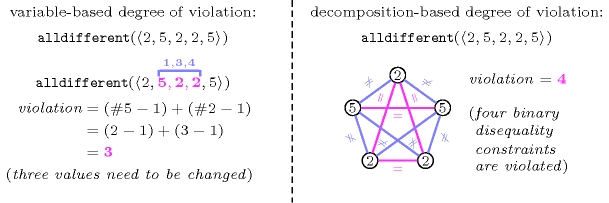### 2.1.5. Cost violation view

Considering a constraint for which all variables are fixed such that the constraint does not hold, a question is to evaluate the degree of violation of that constraint assuming that for a satisfied constraint the degree of violation is equal to zero.

For the $\mathrm{𝚊𝚕𝚕𝚍𝚒𝚏𝚏𝚎𝚛𝚎𝚗𝚝}$ constraint we can define its degree of violation by the minimum number of variables to reassign in order to get a solution. This is called the variable-based degree of violation. We can also define its degree of violation by the number of pairs of variables that do not satisfy the disequality constraint. This is called the decomposition-based degree of violation. Figure 2.1.4 illustrates these two degree of violation costs.

##### Figure 2.1.4. Illustration of the variable-based and the decomposition-based degrees of violation for $\mathrm{𝚊𝚕𝚕𝚍𝚒𝚏𝚏𝚎𝚛𝚎𝚗𝚝}$$\left(〈2,5,2,2,5〉\right)$, where $#v$ denotes the number of occurrences of value $v$ in the argument of the $\mathrm{𝚊𝚕𝚕𝚍𝚒𝚏𝚏𝚎𝚛𝚎𝚗𝚝}$ constraint instance